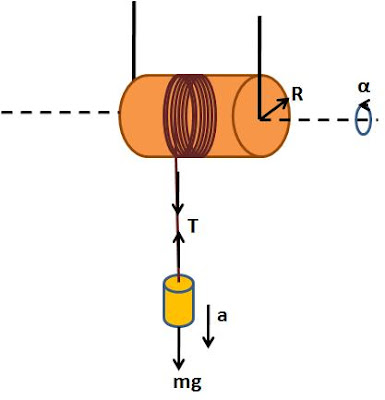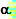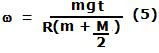## Tuesday, November 11, 2008

### Irodov Problem 1.243Let the tension in the string holding mass m be T. Suppose that the acceleration with which the mass m falls down is given by a. Also suppose that the angular acceleration of the cylindrical pulley about its axis of rotation is given by.

There are two forces acting on mass m in the vertical direction, i) the tension in the string T pulling it up and ii) the force of gravity mg pulling it down. So from Newton's laws we have,Now let us consider the cylinder. The tension in the string creates a torque of TR on the cylinder and causes it to rotate about its axis of rotation and induces the angular acceleration. So if I is the moment of inertia of the cylinder we have,The linear acceleration of the mass m and the angular acceleration of the cylinder are however related to each other as,since the thread is not slipping over the cylinder.

Now solving for a from (1), (2) and (3) we get,a) The angular velocity of the cylinder as a function of time is thus given by,b) If v is the velocity of the mass m at some time t. The kinetic energy of the whole system is given by,